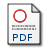# A Parallel Multidimensional Weighted Histogram Analysis Method

Potgieter, Andrew (2014) A Parallel Multidimensional Weighted Histogram Analysis Method, MSc.PDF whaj-gpu-thesis-final.pdf Download (5MB)

## Abstract

The Weighted Histogram Analysis Method (WHAM) is a technique used to calculate free energy from molecular simulation data. WHAM recombines biased distributions of samples from multiple Umbrella Sampling simulations to yield an estimate of the global unbiased distribution. The WHAM algorithm iterates two coupled, non-linear, equations, until convergence at an acceptable level of accuracy. The equations have quadratic time complexity for a single reaction coordinate. However, this increases exponentially with the number of reaction coordinates under investigation, which makes multidimensional WHAM a computationally expensive procedure. There is potential to use general purpose graphics processing units (GPGPU) to accelerate the execution of the algorithm. Here we develop and evaluate a multidimensional GPGPU WHAM implementation to investigate the potential speed-up attained over its CPU counterpart. In addition, to avoid the cost of multiple Molecular Dynamics simulations and for validation of the implementations we develop a test system to generate samples analogous to Umbrella Sampling simulations. We observe a maximum problem size dependent speed-up of approximately 19 for the GPGPU optimized WHAM implementation over our single threaded CPU optimized version. We find that the WHAM algorithm is amenable to GPU acceleration, which provides the means to study ever more complex molecular systems in reduced time periods.

Item Type: Electronic thesis or dissertation (MSc) WHAM, parallel algorithm,free energy calcualtions Software and its engineeringSoftware and its engineering > Software notations and toolsApplied computing > Physical sciences and engineeringComputing methodologies > Modeling and simulation 19 Nov 2014 10 Oct 2019 15:32 http://pubs.cs.uct.ac.za/id/eprint/986View Item